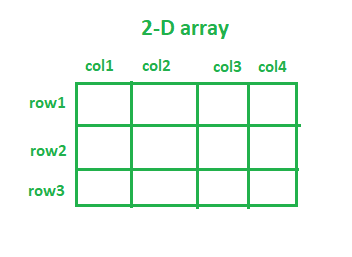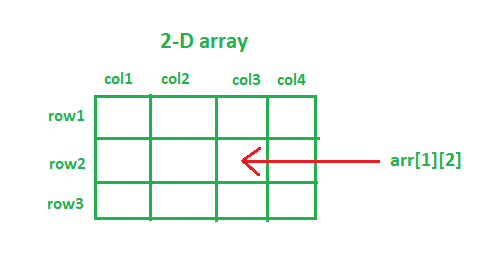# Different Ways To Declare And Initialize 2-D Array in Java

• Difficulty Level : Basic
• Last Updated : 29 Oct, 2021

An array with more than one dimension is known as a multi-dimensional array. The most commonly used multi-dimensional arrays are 2-D and 3-D arrays. We can say that any higher dimensional array is basically an array of arrays. A very common example of a 2D Array is Chess Board. A chessboard is a grid containing 64 1×1 square boxes. You can similarly visualize a 2D array. In a 2D array, every element is associated with a row number and column number. Accessing any element of the 2D array is similar to accessing the record of an Excel File using both row number and column number. 2D arrays are useful while implementing a Tic-Tac-Toe game, Chess, or even storing the image pixels.### Declaring of the 2-D array in Java:

Any 2-dimensional array can be declared as follows:

Syntax:

`data_type array_name[][];   (OR)     data_type[][] array_name;`
• data_type: Since Java is a statically-typed language (i.e. it expects its variables to be declared before they can be assigned values). So, specifying the datatype decides the type of elements it will accept. e.g. to store integer values only, the data type will be declared as int.
• array_name: It is the name that is given to the 2-D array. e.g. subjects, students, fruits, department, etc.

Note: We can write [ ][ ] after data_type or we can write [ ][ ] after array_name while declaring the 2D array.

## Java

 `// java program showing declaration of arrays ` `import` `java.io.*; ` ` `  `class` `GFG { ` `    ``public` `static` `void` `main(String[] args) ` `    ``{ ` ` `  `        ``int``[][] integer2DArray; ``// 2D integer array ` `        ``String[][] string2DArray; ``// 2D String array ` `        ``double``[][] double2DArray; ``// 2D double array ` `        ``boolean``[][] boolean2DArray; ``// 2D boolean array ` `        ``float``[][] float2DArray; ``// 2D float array ` `        ``double``[][] double2DArray; ``// 2D double array ` `    ``} ` `}`

### Initialization of 2-D array in Java:

`data_type[][] array_Name = new data_type[no_of_rows][no_of_columns];`

The total elements in any 2D array will be equal to (no_of_rows) * (no_of_columns).

• no_of_rows: The number of rows an array can store. e.g. no_of_rows = 3, then the array will have three rows.
• no_of_columns: The number of rows an array can store. e.g. no_of_columns = 4, then the array will have four columns.

The above syntax of array initialization will assign default values to all array elements according to the data type specified.

Let us see various approaches of initializing 2D arrays:

## Java

 `// java program to initialize a 2D array ` `import` `java.io.*; ` ` `  `class` `GFG { ` `    ``public` `static` `void` `main(String[] args) ` `    ``{ ` `        ``// Declaration along with initialization ` `        ``// 2D integer array with 5 rows and 3 columns ` `        ``// integer array elements are initialized with 0 ` `        ``int``[][] integer2DArray = ``new` `int``[``5``][``3``]; ` `        ``System.out.println( ` `            ``"Default value of int array element: "` `            ``+ integer2DArray[``0``][``0``]); ` ` `  `        ``// 2D String array with 4 rows and 4 columns ` `        ``// String array elements are initialized with null ` `        ``String[][] string2DArray = ``new` `String[``4``][``4``]; ` `        ``System.out.println( ` `            ``"Default value of String array element: "` `            ``+ string2DArray[``0``][``0``]); ` ` `  `        ``// 2D boolean array with 3 rows and 5 columns ` `        ``// boolean array elements are initialized with false ` `        ``boolean``[][] boolean2DArray = ``new` `boolean``[``4``][``4``]; ` `        ``System.out.println( ` `            ``"Default value of boolean array element: "` `            ``+ boolean2DArray[``0``][``0``]); ` ` `  `        ``// 2D char array with 10 rows and 10 columns ` `        ``// char array elements are initialized with ` `        ``// '\u0000'(null character) ` `        ``char``[][] char2DArray = ``new` `char``[``10``][``10``]; ` `        ``System.out.println( ` `            ``"Default value of char array element: "` `            ``+ char2DArray[``0``][``0``]); ` ` `  `        ``// First declaration and then initialization ` `        ``int``[][] arr; ``// declaration ` ` `  `        ``// System.out.println("arr: "+ arr); ` `        ``// The above line will throw an error, as we have ` `        ``// only declared the 2D array, but not initialized ` `        ``// it. ` `        ``arr = ``new` `int``[``5``][``3``]; ``// initialization ` `        ``System.out.println(``"arr: "` `+ arr[``0``][``0``]); ` `    ``} ` `}`

Note: When you initialize a 2D array, you must always specify the first dimension(no. of rows), but providing the second dimension(no. of columns) may be omitted.

In the code snippet below, we have not specified the number of columns. However, the Java compiler is smart enough to manipulate the size by checking the number of elements inside the columns.

## Java

 `import` `java.io.*; ` ` `  `class` `GFG { ` `    ``public` `static` `void` `main(String[] args) ` `    ``{ ` `        ``// The line below will throw an error, as the first ` `        ``// dimension(no. of rows) is not specified ` `        ``int``[][] arr = ``new` `int``[][``3``]; ` ` `  `        ``// The line below will execute without any error, as ` `        ``// the first dimension(no. of rows) is specified ` `        ``int``[][] arr = ``new` `int``[``2``][]; ` `    ``} ` `}`

You can access any element of a 2D array using row number and column number.### Approach 2

In the code snippet below, we have not specified the number of rows and columns. However, the Java compiler is smart enough to manipulate the size by checking the number of elements inside the rows and columns.

## Java

 `import` `java.io.*; ` ` `  `class` `GFG { ` `    ``public` `static` `void` `main(String[] args) ` `    ``{ ` `        ``String[][] subjects = { ` `            ``{ ``"Data Structures & Algorithms"``, ` `              ``"Programming & Logic"``, ``"Software Engineering"``, ` `              ``"Theory of Computation"` `},                       ``// row 1 ` ` `  `            ``{ ``"Thermodynamics"``, ``"Metallurgy"``, ` `              ``"Machine Drawing"``, ` `              ``"Fluid Mechanics"` `},                             ``// row2 ` ` `  `            ``{ ``"Signals and Systems"``, ``"Digital Electronics"``, ` `              ``"Power Electronics"` `}                            ``// row3 ` `        ``}; ` ` `  `        ``System.out.println( ` `            ``"Fundamental Subject in Computer Engineering: "` `            ``+ subjects[``0``][``0``]); ` `        ``System.out.println( ` `            ``"Fundamental Subject in Mechanical Engineering: "` `            ``+ subjects[``1``][``3``]); ` `        ``System.out.println( ` `            ``"Fundamental Subject in Electronics Engineering: "` `            ``+ subjects[``2``][``1``]); ` `    ``} ` `}`

### Approach 3

Moreover, we can initialize each element of the array separately. Look at the code snippet below:

## Java

 `import` `java.io.*; ` `import` `java.util.*; ` ` `  `class` `GFG { ` `    ``public` `static` `void` `main(String[] args) ` `    ``{ ` `        ``int``[][] scores = ``new` `int``[``2``][``2``]; ` `        ``// Initializing array element at position, ` `        ``// i.e. 0th row and 0th column ` `        ``scores[``0``][``0``] = ``15``; ` `        ``// Initializing array element at position, ` `        ``// i.e. 0th row and 1st column ` `        ``scores[``0``][``1``] = ``23``; ` `        ``// Initializing array element at position, ` `        ``// i.e. 1st row and 0th column ` `        ``scores[``1``][``0``] = ``30``; ` `        ``// Initializing array element at position, ` `        ``// i.e. 1st row and 1st column ` `        ``scores[``1``][``1``] = ``21``; ` ` `  `        ``// printing the array elements individually ` `        ``System.out.println(``"scores = "` `                           ``+ scores[``0``][``0``]); ` `        ``System.out.println(``"scores = "` `                           ``+ scores[``0``][``1``]); ` `        ``System.out.println(``"scores = "` `                           ``+ scores[``1``][``0``]); ` `        ``System.out.println(``"scores = "` `                           ``+ scores[``1``][``1``]); ` `        ``// printing 2D array using Arrays.deepToString() method ` `        ``System.out.println( ` `            ``"Printing 2D array using Arrays.deepToString() method: "``); ` `        ``System.out.println(Arrays.deepToString(scores)); ` `    ``} ` `}`

### Approach 4

Using the above approach for array initialization would be a tedious task if the size of the 2D array is too large. The efficient way is to use for loop for initializing the array elements in the case of a large 2D array.

## Java

 `import` `java.io.*; ` ` `  `class` `GFG { ` `    ``public` `static` `void` `main(String[] args) ` `    ``{ ` `        ``int` `rows = ``80``, columns = ``5``; ` `        ``int``[][] marks = ``new` `int``[rows][columns]; ` ` `  `        ``// initializing the array elements using for loop ` `        ``for` `(``int` `i = ``0``; i < rows; i++) { ` `            ``for` `(``int` `j = ``0``; j < columns; j++) { ` `                ``marks[i][j] = i + j; ` `            ``} ` `        ``} ` ` `  `        ``// printing the first three rows of marks array ` `        ``System.out.println(``"First three rows are: "``); ` `        ``for` `(``int` `i = ``0``; i < ``3``; i++) { ` `            ``for` `(``int` `j = ``0``; j < columns; j++) { ` `                ``System.out.printf(marks[i][j] + ``" "``); ` `            ``} ` `            ``System.out.println(); ` `        ``}         ` `    ``} ` `}`

We can use arr. length can be used to find the size of the rows (1st dimension), and arr.length can be to find the size of the columns (2nd dimension).

### Approach 5: (Jagged arrays)

There may be a certain scenario where you want every row to a different number of columns. This type of array is called a Jagged Array.

## Java

 `import` `java.io.*; ` ` `  `class` `GFG { ` `    ``public` `static` `void` `main(String[] args) ` `    ``{ ` `        ``// declaring a 2D array with 2 rows ` `        ``int` `jagged[][] = ``new` `int``[``2``][]; ` ` `  `        ``// not specifying the 2nd dimension, ` `        ``// and making it as jagged array ` `        ``// first row has 2 columns ` `        ``jagged[``0``] = ``new` `int``[``2``]; ` `        ``// second row has 2 columns ` `        ``jagged[``1``] = ``new` `int``[``4``]; ` `        ``// Initializing the array ` `        ``int` `count = ``0``; ` `        ``for` `(``int` `i = ``0``; i < jagged.length; i++) { ` `            ``// remember to use jagged[i].length instead of ` `            ``// jagged.length, since every row has ` `            ``// different number of columns ` `            ``for` `(``int` `j = ``0``; j < jagged[i].length; j++) { ` `                ``jagged[i][j] = count++; ` `            ``} ` `        ``} ` ` `  `        ``// printing the values of 2D Jagged array ` `        ``System.out.println(``"The values of 2D jagged array"``); ` `        ``for` `(``int` `i = ``0``; i < jagged.length; i++) { ` `            ``for` `(``int` `j = ``0``; j < jagged[i].length; j++) ` `                ``System.out.printf(jagged[i][j] + ``" "``); ` `            ``System.out.println(); ` `        ``} ` `    ``} ` `}`

Implementation:

Let’s look at a simple program to add two 2D arrays:

## Java

 `import` `java.io.*; ` `import` `java.util.*; ` ` `  `class` `GFG { ` `    ``public` `static` `void` `main(String[] args) ` `    ``{ ` `        ``int``[][] arr1 = { { ``1``, ``2``, ``3` `}, { ``4``, ``5``, ``6` `} }; ` `        ``int``[][] arr2 = { { ``4``, ``5``, ``6` `}, { ``1``, ``3``, ``2` `} }; ` `        ``int``[][] sum = ``new` `int``[``2``][``3``]; ` ` `  `        ``// adding two 2D arrays element-wise ` `        ``for` `(``int` `i = ``0``; i < arr1.length; i++) { ` `            ``for` `(``int` `j = ``0``; j < arr1[``0``].length; j++) { ` `                ``sum[i][j] = arr1[i][j] + arr2[i][j]; ` `            ``} ` `        ``} ` `         `  `        ``System.out.println(``"Resultant 2D array: "``); ` `        ``for` `(``int` `i = ``0``; i < sum.length; i++) { ` `            ``System.out.println(Arrays.toString(sum[i])); ` `        ``} ` `    ``} ` `}`

My Personal Notes arrow_drop_up
Recommended Articles
Page :